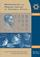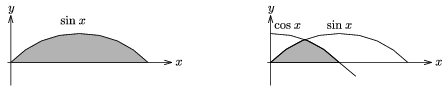Középiskolai Matematikai és Fizikai Lapok
Informatika rovattal
 Már regisztráltál? Új vendég vagy?# Angol nyelvű szám, 2002. december

Előző oldalTartalomjegyzékKövetkező oldalMEGRENDELŐLAP

## Problems in Physics

M. 237. Make a 1.5 m-long line pendulum using a table-tennis ball as the weight. How does the work done by the aerodynamic drag (the energy lost) depend on the starting height h? What is the average power of the aerodynamic force during a half swing?

(6 points)

Suggested by: Varga István, Békéscsaba

P. 3561. According to a car safety advertisement a 50 km/h collision into a wall has the same result as falling off the top of a four-storey building. What storey height did they think of?

(3 points)

Suggested by: Kopcsa József, Debrecen

P. 3562. In a fruit farm the crates are conveyed on steel plates dragged by tractors. The mass of a steel plate is 20 kg and 400 kg of fruit is piled on it. The friction coefficient between the plate and the soil is 0.6. To what extent does the steel plate heat up on a 100 meter road if the internal energy of the steel plate is increased by 40% of the work done by the friction?

(3 points)

Keresd a megoldást!'' competition, Szeged

P. 3563. There are two cars following each other on a narrow straight road. Their speed is 72 km/h each. The leading one begins to brake and stops with constant deceleration while his velocity decreases 5 m/s a second. The response time of the other driver is 1 second, and he can decrease his velocity by 4 m/s a second.

a) What distance should there be at least between the two cars to avoid a collision?

b) If they keep the minimally safe distance, how long will it take them to be at a 22 m distance of each other after the fist one begins to brake?

(4 points)

Suggested by: Szegedi Ervin, Debrecen

P. 3564. How many balloons of a 25 cm diameter can be inflated from a 0.1 m3 volume gas cylinder filled with helium at a pressure of 1.5x107 Pa? The gas pressure in a balloon rises to 1.02x105 Pa when the temperature of the gas reaches the temperature of the environment. The gas cylinder has been in the place where the balloons are filled for days.

(4 points)

Suggested by: Hilbert Margit, Szeged

P. 3565. We want to cool down 15 l of 80 oC water to 25 oC by putting 0 oC ice into it. What minimum volume should the vessel have to prevent the water from overflowing at the rim?

(3 points)

Suggested by: Holics László, Budapest

P. 3566. We want to throw a weight of 5 kg over a 1.5 m high wall, starting from ground level at a 2 m distance from the wall.

a) what should the direction of the 8 m/s initial velocity be to throw the weight the furthest beyond the wall? What is this distance?

b) What is the lowest energy to use for throwing the weight over the wall? What is the direction of the initial velocity in this case?

(5 points)

Suggested by: Szűcs József, Pécs

P. 3567. Determine the size of the two hatched areas in the figure without using the integral calculus, by some simple physical reasoning.(5 points)

Suggested by: Sári Péter, Budapest

P. 3568. We fix a line pendulum with a pointlike weight of mass m to a horizontal metal ceiling. The period of the pendulum for small amplitudes is T1. Then we put some electric charge on the weight and make it swing with a small amplitude again. The line is insulating. The period now is T2. What is the charge put on the weight? (The data: m= 5 g, T1=2.02 s, T2=2.03 s, g=9.81 m/s2.)

(5 points)

Suggested by: Légrádi Imre, Sopron

P. 3569. There is a 1.12 A electric current going through a working discharge tube filled with hydrogen gas. How many electrons pass the cross-section of the tube in one second if the number of electrons leaving the cathode is 3x1018 a second?

(4 points)

Increasing the inductivity further, the voltage of the coil reaches a maximum of Umax , and then begins to decrease. Determine the Umax /U0 ratio and calculate its value when $\displaystyle R=2\sqrt{2}X_C$.